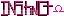-=+=- -=+=- -=+=- -=+=- -=+=- -=+=- -=+=- -=+=- -=+=- -=+=- -=+=- -=+=- -=+=- -=+=- -=+=- -=+=- -=+=- -=+=- -=+=- -=+=- -=+=- -=+=- -=+=- -=+=- -=+=- -=+=- -=+=- -=+=- -=+=- -=+=- (c) WidthPadding Industries 1987 0|408|0 -=+=- -=+=- -=+=- -=+=- -=+=- -=+=- -=+=- -=+=- -=+=- -=+=- -=+=- -=+=- -=+=- -=+=- -=+=- -=+=- -=+=- -=+=- -=+=- -=+=- -=+=- -=+=- -=+=- -=+=- -=+=- -=+=- -=+=- -=+=- -=+=- -=+=-
SoCoder -> Snippet Home -> Website Development

StickyCreated : 04 January 2010

System : Nintendo Wii
Language : Flash

### Integer\\IP converter

Converts between integers and IPs

Just two functions I wrote that convert an IP to an integer or vice versa. Useful for inserting into databases as an int only needs four bytes whereas a text representation of an IP can need up to 15.

This one converts from an integer into an IP.

And this does the reverse, converting an IP to an integer.

### Comments

Tuesday, 05 January 2010, 00:27
Jayenkai*wonders why you used powers rather than bitshifting.*

Nice and simple.
This is one of those really simple space saving methods that everyone seems to completely forget about.Tuesday, 05 January 2010, 04:09
StickyI used powers instead of bitshifting because I couldn't work out how to force it as an unsigned int - as soon as I bitshifted 24 it made it negative because it was signed. So, powers were just easier in the end.Tuesday, 05 January 2010, 04:20
JayenkaiOh yeah.. hmm.. *shrugs*Wednesday, 06 January 2010, 20:11
SchererererYour comment about negative numbers appearing in your integer inspired me to write a quick article on the way signed numbers are stored in a computer.

www.socoder.net/index.php?article=21718

hope it helps! (particularly the comments on overflow)